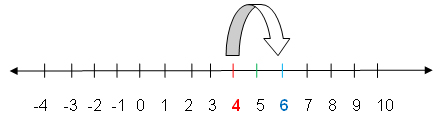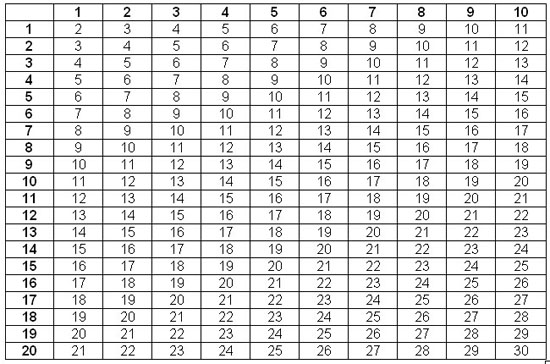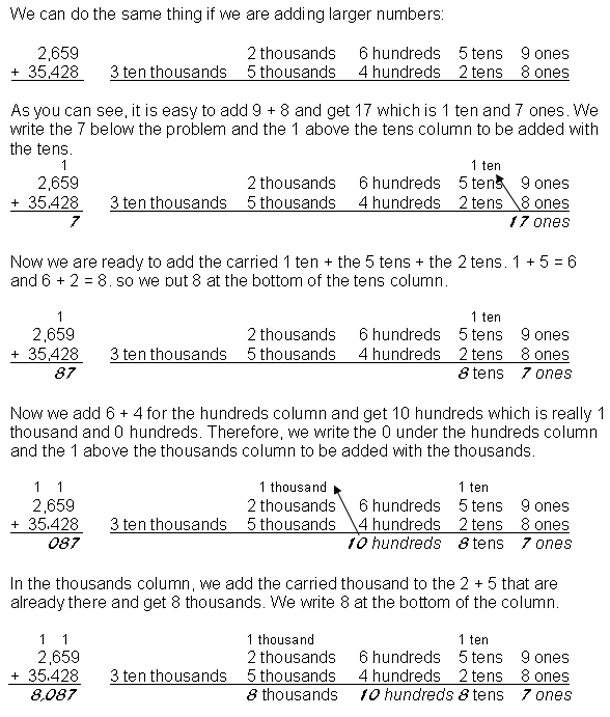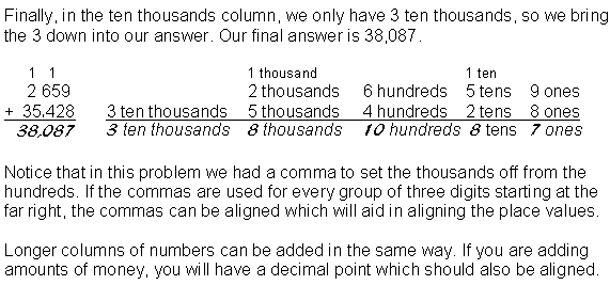Math Skills: How to Do Addition

Quite simply, addition is putting two or more things or groups of things together. In other words, if there are 4 boys and 2 girls going to class, how many young people are going to class?
Our first method of figuring out how many there are is to count them.
When we plot our counting like this, it looks similar to the number line for addition.
 Movement on a Number Line

On the number line, we locate the number 4 (for the 4 boys) and then we move to the right 2 ticks to add the 2 girls. The number on which we land is 6 which is the same number we got when we counted.Grouping of Objects
With the boys and girls we had two groups. There are times when we can group objects and count them, but if we have more than 10 in a group or more than 3 or 4 groups, this becomes difficult. It is then easy to lose track of our counting.

If the objects are not all in the same place at the same time, it is nearly impossible to group and count them. However, we can count the number in each group and then add the numbers from all the groups.

In many cases we do not know or care what the objects are, where they are, or what color they are. We simply need to add the numbers together.

Each of the numbers that is being added is called an addend and the answer is called a sum. The type of sign we use to indicate addition is a ‘plus sign' which looks like this: +.

4 + 7 =

Or we can show it vertically:

3
+ 5
_____

When we show it horizontally, we always place the plus sign between each pair of addends.

2 + 3 + 5 + 7 =

When we are adding three or more numbers and have them lined up by place value vertically, the plus sign is not always necessary.

Interested in learning more? Why not take an online Basic Math course?

425
21
13
110
_____

Lining the numbers up by place value vertically is usually an easier way to add numbers. Notice that a line goes below the last addend to indicate that the numbers are ready to be added.

Adding becomes easier and quicker if we memorize some addition facts. The facts given on the table below are one through ten in one direction (left to right) and one through twenty in the other direction (top to bottom).

To add 7 + 8, you can locate the 7 along the top and the 8 along the left side, then trace the lines of cells until they intersect. The number in that cell is the answer. In this case it is 15. Alternatively, you could have located the 7 along the left and the 8 along the top and found their intersection. It should also be 15.

By memorizing the facts for 1 – 10, your life will become much easier. You will be able to quickly add a group of numbers simply by looking at them.Flash cards are a great aid in learning addition facts. Use online flash cards at:

425        4 hundreds        2 tens           5 ones
21                                   2 tens           1 one
13                                   1 ten             3 ones
110         1 hundred         1 ten             0 ones

In this way we start by adding the ones column: 5 + 1 = 6 and 6 + 3 = 9 and 9 + 0 = 9. We place the 9 below the ones column.

425
21
13
110
9

Then we add the tens column: 2 + 2 = 4 and 4 + 1 = 5  and 5 + 1 = 6. We place the 6 below the tens column.

425
21
13
110
69

Finally, we add the hundreds column: 4 + 1 = 5. And we place the 5 below the hundreds column. This gives us a final answer of 569 (five hundred sixty nine).

425
21
13
110
569

It is essential that we are careful to line up the digits by place value when the sum of a column is ten or greater. If we add 3 + 9, we can simply write 12. However, if we add

13 + 19

we will not write 12 and 20 or 12 and 2 as that would not make any sense.

By writing the problem vertically, we can understand where and how to write the answer:

13                1 ten         3 ones
+ 19                1 ten         9 ones

When we add the 3 ones and the 9 ones, we get 12 ones which is 1 ten and 2 ones.

13                1 ten         3 ones
+ 19                1 ten         9 ones
12 ones
Or:                             1 ten          2 ones

We will carry the 1 ten into the tens column, giving us 1 + 1 + 1 in that column:

1 ten
13                1 ten         3 ones
+ 19                1 ten         9 ones
2 ones

That gives us 3 tens: 3 tens

So our answer is 3 tens and 2 ones, or 32:

13
+ 19
_______
32

Essentially, our answer is 12 ones and 2 tens or 20, but the 12 and the 20 would still have to be added to get our final answer of 32.